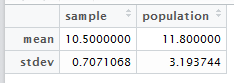Select Page

# A

Import vector of values of ice cream purchase numbers

>a <- c(8, 14, 16, 10, 11)

Generate random sample of 2 values and save to a vector

> b <- sample(a,2)
 10 11

Calculate mean and standard deviation of the sample.

>mean(b)
 10.5
> sd(b)
 0.7071068

Create data.frame out of mean and stdev for the sample and population.

mean(a)
 11.8
> sd(a)
 3.193744

smp <- c(10.5,0.7071068)
> pop <- c(11.8,3.193744)
> c <- data.frame < (smp, pop)# B

1. I think that the sample proportion will have the approximately the same distribution since nq = 5.
2. I think 100 is the smallest value of n for which p is approximately normal because anything smaller than n = 100 will make np < 5.  The high value of p  is very limiting.